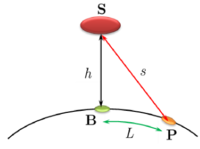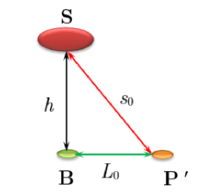# Distance to satellite base along the earth

#### Brotherbobby

##### New memberStatement of the Problem : A satellite located at a height $$\displaystyle h$$ from the base B sends radio signals to your place P. These signals give us the distance $$\displaystyle s$$ to your place up to a certain measurement error $$\displaystyle \delta s$$. Your place P is located at a distance $$\displaystyle L$$ along the earth, as shown in the diagram above. Use the methods of differential calculus to calculate $$\displaystyle L$$ to a first approximation.Solution : I Imagine a simplified triangular image of the three points as shown above. The distance BP' = $$\displaystyle L_0 = \sqrt{s_0^2 - h^2}$$. The original distance $$\displaystyle L = L_0 +\delta L \approx L_0 + \left( \frac{dL}{ds}\right)_{s_0}\delta s$$.

From the triangle above, using the methods of trigonometry and calculus, we get $$\displaystyle \frac{dL}{ds} = \frac{s}{L}$$ and hence $$\displaystyle \left( \frac{dL}{ds}\right )_{s_0} = \frac{s_0}{L_0} \text{, hence the original distance}\; L = L_0 + \frac{s_0}{L_0} \delta s \Rightarrow \boxed{L = L_0\left( 1+\frac{s_0}{L_0^2}\delta s \right)}$$.

The satellite gives the error in the measurement of $$\displaystyle s$$ which is $$\displaystyle \delta s$$ and so the distance L can be found.

Is my working correct?

#### tkhunny

##### Moderator
Staff member
Seems as though you are allowing for only the measurement error and ignoring the curvature of the earth.

#### Dr.Peterson

##### Elite Member
View attachment 12846

Statement of the Problem : A satellite located at a height $$\displaystyle h$$ from the base B sends radio signals to your place P. These signals give us the distance $$\displaystyle s$$ to your place up to a certain measurement error $$\displaystyle \delta s$$. Your place P is located at a distance $$\displaystyle L$$ along the earth, as shown in the diagram above. Use the methods of differential calculus to calculate $$\displaystyle L$$ to a first approximation.
The problem confuses me, so I'm not entirely sure what you are expected to do. It might help if you tell us what topics you have been learning recently that this might be intended to exercise. Have you seen examples that include a measurement error?

One problem is that the measurement error is irrelevant to actually calculating the value of L. All it can be used for is to determine the likely error in your value of L. Might that be what they mean by "methods of differential calculus" -- error propagation?

Secondly, nothing indicates that you are to assume a flat earth, so I very much doubt that what you did is what they want; on the other hand, they didn't mention what to use for the radius of the earth. But it is not hard to make a formula for L using geometry/trigonometry (the Law of Cosines, for example). No calculus is needed. That gives a "first approximation", and the measurement error can tell how close that approximation is. That's the only way I see to get a "first approximation"